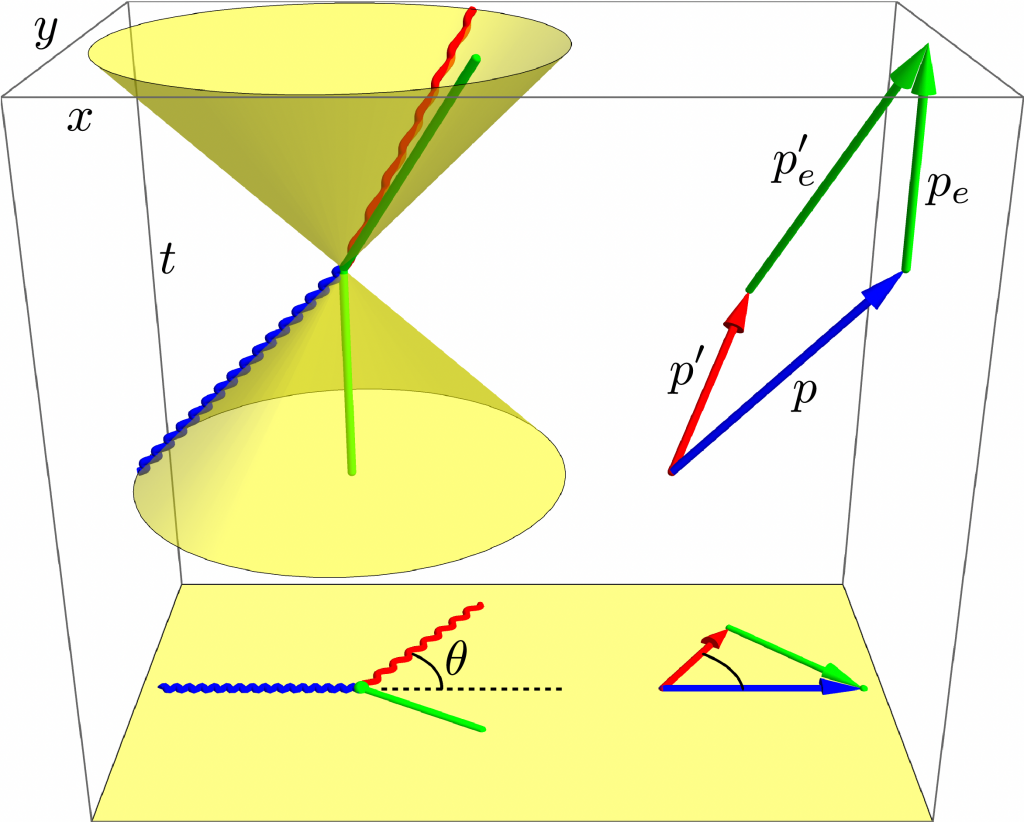# A Century of Compton Scattering

One hundred years ago today the Physical Review published research on light scattering electrons that would earn its author, Wooster graduate Arthur Compton, a Physics Nobel Prize.

By relativistically conserving spacetime momentum, as in the diagram below, and treating light as particles now called photons, Compton discovered that deflecting an electron through an angle $\theta$ stretches the light’s wavelength by

$$\frac{ \Delta \lambda}{\lambda_c} = 1 - \cos\theta,$$

where $\lambda_c = h / mc \sim 1/40 \textup{~\AA}$ is the Compton wavelength of the electron. Compton’s experiment helped convince the physics community of wave-particle duality. Today, sophomores Compton scatter in our Modern Physics lab.Compton scattering of a photon (blue to red) from an electron (green) in space (bottom) and spacetime (top). The photon lives on the light cones while the electron lives in the light cones.